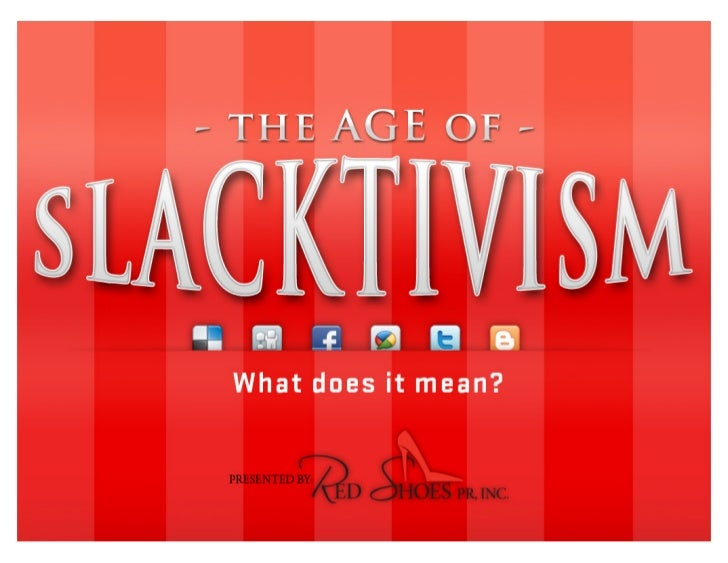Successfully reported this slideshow.
We use your LinkedIn profile and activity data to personalize ads and to show you more relevant ads. You can change your ad preferences anytime.Upcoming SlideShare
×

The Age of Slacktivism Motivating the Masses

1,910 views

Published on

The world of giving for individual donors is changing. It is now more important than ever to reach the masses and make it easy for them to give and share your cause. In this presentation, Red Shoes PR breaks down this new trend and how you can tap into the power of the slacktivist.

Published in: Education
• Full Name
Comment goes here.

Are you sure you want to Yes No
Your message goes here• Be the first to comment

The Age of Slacktivism Motivating the Masses

1. 1. !"#\$%&(()*++,-\$.",(/"\$0(!  1,#+*-\$,#2,/(-"#\$%&(( (/+,+*\$.",30\$45.627(!  !"(-\$*-(-82,52,/(#29+*+,-0%(!  :++(;8\$-("-8+*(,",<)*"=-(( \$*+(#"2,/(!  >+-+*72,+(%"?*(( )"-+,.\$0(
2. 2. @A\$7)0+("B(0\$45.627( !   !+A.,/(>",\$.",( !   :2/,2,/(2,-+*,+-()+..",( !   !8+(;+\$*2,/("B(;*2-C\$,#( D\$;\$*+,+(C*\$4+0+-E(( !   F?G,/(\$(*2CC",(7\$/,+-(",(\$(6+8240+( !   H"2,2,/(\$(I\$4+C""5(/*"?)J()".,/(( 2?+<"*2+,-+#(K"?!?C+(62#+"J(( !   !\$52,/()\$*-(2,(8"*-<-+*7(C"%4"L(( ?48(\$(M?%(N"-82,/(>\$%("*(( @\$*-8(O"?*P(
3. 3. Q8\$-(2(0\$45.627RR(!  !"#\$%&()*(D\$44"*#2,/(-"(Q252)+#2\$E(2(\$( B"*7+#("?-("B(-8+(;"*#(!"#\$%&(\$,#(#\$()*!+P(!  >+4*2C+(SB++0</""#S(7+\$?*+J(2,(?))"*-("B( \$,(2?+("*("42\$0(4\$?+J(-8\$-(8\$6+(02L0+("*(,"(((( (((((((((((((()*\$4.4\$0(+9+4-("-8+*(-8\$,(-"(7\$5+(-8+(( (((((((((((((()+*",(#"2,/(2-(B++0(\$.B\$4.",P(!8+(( ((((((((((((((\$4-(\$0"(-+,#(-"(*+T?2*+(02L0+()+*",\$0(( ((((((((((((((+9"*-(B*"7(-8+(0\$45.62-P(
4. 4. Q8\$-(2(\$(U2.V+,(F820\$,-8*")2-R(!  I"*(-8+("42\$0( 7+#2\$(+7)";+*+#( #","*J( ++2,/(#2*+4-( 27)\$4-(\$,#(C?20#2,/( *+0\$.",82)( C+4"7+(-8+( )*2"*2-%P( D\$44"*#2,/(-"(W+"9(X262,/-",J( Y\$8\$C0+<:"42\$0(W""#E((
5. 5. !  Z:"42\$0(7+#2\$(8\$(/26+,(6"24+(-"( -8+(2,#262#?\$0()820\$,-8*")2-(\$,#( -8+*+(2(\$(/*+\$-(#+\$0("B(/""#(-8\$-( 4\$,(4"7+(B*"7(")+,2,/(?)(-8+( )*"4+("B()820\$,-8*")%(\$,#( 8+0)2,/(*+#+=,+(;8\$-(2-(7+\$,(-"( 48\$7)2",(\$(4\$?+(%"?()+*",\$00%( 4\$*+(\$C"?-P[(( –  \$2#(\$*2(>?,,(:\$*\$-"65%J(]24+( F*+2#+,-("B(:"42\$0(^,,"6\$.",(\$-(-8+( U\$+(I"?,#\$.",P((
6. 6. !  Z!8+(2,4*+\$2,/()")?0\$*2-%("B(( ",02,+(B?,#*\$22,/(4"7)+..",(( \$,#(4",-+-(2(\$0"(\$(-+-\$7+,-(( -"(-8+(2,4*+\$2,/(27)"*-\$,4+("B(-8+(( ((((((2,#262#?\$0()820\$,-8*")2-P[(
7. 7. >",&-(-8*";("?-(-8+( "0#(;\$%("B(B?,#*\$22,/(( _?-(%+-`(!  :"42\$0(7+#2\$(\$,#(",02,+(/262,/( ?))"*-(%"?*("6+*\$00(+9"*-(!  U8\$,/2,/(-8+(72A("B(?))"*-+*(!  K"?*("*/\$,2V\$.",(,";(8\$(-8+( )"-+,.\$0(-"(-\$)(2,-"(\$(;8"0+(,+;( 7\$*5+-("B(#","*`(
8. 8. a8(-8"+(0\$45.62-`( !  H?-(C+4\$?+(-82(-%)+("B(#","*(( 025+(-"(/26+(2,(-8+(+\$2+-(( ;\$%()"2C0+(#"+,&-(7+\$,(2-( 2,&-(/""#( K"?(,";(8\$6+(\$6\$20\$C0+(-""0( -"(*+\$48(\$(0\$*/+*(\$?#2+,4+(2,(\$( 6+*%(+b42+,-(7\$,,+*( Remember, it’s relationship building!!
9. 9. >",&-("6+*-82,5(",02,+(*+0\$.",82)( C?20#2,/(!  K"?(2,-+*\$4-(;2-8("7+",+(B"*(\$(=*-(.7+(%"?( 7\$5+(2,-*"#?4.",(!  K"?(+,/\$/+(;2-8(-8+("-8+*()+*",(!  !;"(4"7)",+,-(-"(+,/\$/+7+,-c(( -\$052,/(\$,#(02-+,2,/(!  !82,5(\$C"?-(/+G,/(-8+7(2,(%"?*(#""*("*(-"( 622-(%"?*(;+C2-+(
10. 10. M?-(/26+(4\$*+B?0(4",2#+*\$.",(-"(d(!  O";(\$*+(%"?(/"2,/(-"(\$))+\$0(-"(\$(0\$45.62-(\$,#( 7\$5+(+,/\$/+7+,-J(?))"*-(\$,#(#",\$.",(+\$%R(!  Y\$5+(-8+()*"4+(-?*,5+%(!  I"*/+-(F>I(!  W"(B"*(",02,+(+\$%(2/,<?)(!  Y"C20+(/262,/(!  W26+(-8+7("7+-82,/(-"(-\$05(( \$C"?-38";(-8+2*(?))"*-(
11. 11. !  U0245(\$,#(W26+(!  K"?*(;+C2-+(2(%"?*(",02,+(B*",-(#""*(!  Y?48(025+(%"?*(C*245(\$,#(( 7"*-\$*(B*",-(#""*J(8";(#"(( %"?(;+04"7+(#","*(-"(( %"?*(;+C2-+R(!  !82,5(025+(%"?*(#","*J(( (;8\$-(\$*+(-8+2*(,++#(\$,#(;\$,-R(
12. 12. Q8%(O\$2.(B?,#*\$22,/(;"*5+#( !  ^-(;\$(7\$#+(+\$%( !  Y"C20+(!+48,"0"/%( !  !+A.,/(
13. 13. e+?0-R(!   Y"*+(-8\$,(fgh(72002",(*\$2+#(!   !8+(4+,-+*(\$2#("B(-8"+(i7+*24\$,(;8"( 8\$6+(#",\$-+#(7",+%(-"(8+0)(O\$2.J(jkl( \$%(-8+%(/\$6+(7",+%(62\$(-+A-(7+\$/+J( 4"7)\$*+#(-"(jml(C%(-+0+)8",+P((!   N+\$*0%(mgl(\$2#(-8+%(#",\$-+#(62\$(-8+( Q+CJ(\$,#(nl(#2#("(C%(+<7\$20P(DF+;( e++\$*48E(!   gol(\$%(-8+%(/\$6+(2,()+*",J(( ?48(\$(\$-(\$(48?*48(
14. 14. iC"?-(B*++*24+P4"7(+,--.(\$-/0#)/123/43#")5(!  F*"62#+(+#?4\$.",(( -"(+6+*%",+(B"*(( B*++P(!  O+0)(+,#(;"*0#(( 8?,/+*(C%()*"62#2,/(( *24+(-"(8?,/*%(( )+")0+(B"*(B*++P(
15. 15. I*++*24+P4"7c(O";(-"()0\$%(!  U0245(",(-8+(*2/8-(\$,;+*(2,(-8+(72##0+("B(-8+()\$/+P((!  ^B(%"?(/+-(2-(*2/8-J(%"?(( /+-(\$(8\$*#+*(T?+.",P(( ^B(%"?(/+-(2-(;*",/J(%"?(( /+-(\$,(+\$2+*(T?+.",P((!  I"*(+\$48(\$,;+*(%"?(( /+-(*2/8-J(;+(#",\$-+(( jh(/*\$2,("B(*24+(-"(-8+(( 1,2-+#(N\$.",(( Q"*0#(I""#(F*"/*\$7P((
16. 16. e+?0-(-"(#\$-+R( (((D,+\$*0%(pg(C2002",`E( /*\$2,("B(*24+(8\$6+( C++,(+\$*,+#(-"(#\$-+P( !8\$-(2(+,"?/8(-"( B++#(7"*+(-8\$,(kPg( 72002",()+")0+(B"*( ",+(#\$%`(
17. 17. @)24(W262,/(!8*"?/8(!  @)2448\$,/+P"*/(!  Y22",(( @)24(U8\$,/+( \$7)02=+(-8+( 6"24+(\$,#(27)\$4-( "B(/*\$*""-( 48\$,/+7\$5+*(q( "42\$0( +,-*+)*+,+?*P(
18. 18. >",\$.,/(7\$#+(+\$%(",(( +)2448\$,/+P"*/(!  W""/0+(U8+45"?-(!  F\$%)\$0(!  :2/,(?)(B"*(7",-80%( ?C4*2C+*(#",\$.",(
19. 19. W+G,/(2,6"06+#(7\$#+(+\$%(
20. 20. :++(%"?*(#",\$.",(\$-(;"*5(!  !8*"?/8(\$()*"_+4-( "6+*62+;(!  e+?0-(-"(#\$-+(!  8L)c33 +)2448\$,/+P"*/3 )*"_+4-P)8)(
21. 21. !82,5(%"?*(#","*(\$*+(*+\$#2,/(%"?*( ,+;0+L+*(\$,#(\$,,?\$0(*+)"*-R( !   Q8%(#",&-(%"?(\$5(%"?*(#","*3( ?))"*-+*(8";(-8+%()*+B+*(-"(5++)(?)(-"( #\$-+(;2-8(%"?*("*/\$,2V\$.",( !   M+(;8+*+(-8+%(\$*+(/+G,/(-8+2*( 2,B"*7\$.",( !   !"#\$%&(#","*(2(025+0%(/+G,/(( -8+2*(2,B"*7\$.",(-8*"?/8(\$(6\$*2+-%("B( "?*4+J(2,(\$(6\$*2+-%("B(;\$%( !   F+")0+(\$*+(ohl(7"*+(025+0%(-"(*+)",#(-"( %"?*(4"77?,24\$.",(2B(-8+%(\$*+(*+4+26+#( 2,(-8+(7\$,,+*(-8+%()*+B+*P(r(F+-+*( :8\$,57\$,(
22. 22. !  \$,(",02,+(*+)"*-(C%(-8+( NF>(W*"?)(8";+#(-8\$-( sjl("B(C\$C%<C""7+*( 2,-+*,+-(?+*(2,(-8+(1P:P( 8\$6+(622-+#(2-+(-8\$-( "9+*(-*+\$72,/("*( #";,0"\$#\$C0+(62#+"(!  \$,#(kjl(8\$6+(622-+#(",02,+("42\$0(,+-;"*5P((
23. 23. !  X\$*/+-(B\$-+-(/*";2,/(#+7"/*\$)824(",(( "42\$0(7+#2\$(D?48(\$(I\$4+C""5(\$,#(:5%)+E(( 2(;"7+,J(nn(\$,#("0#+*(!  i44"*#2,/(-"(\$(mhho(F+;(2,-+*,+-(-?#%(d((!  Y"*+(-8\$,(8\$0B("B(\$00(C""7+*(\$*+( (",02,+(",(\$,(\$6+*\$/+(#\$%J(\$,#(( 7"*+(-8\$,(\$(-82*#(/"(",02,+(( +6+*\$0(.7+(\$(#\$%P((!  i,#(kjl("B(C""7+*(/"(",02,+(( _?-(-"(8\$6+(B?,P((
24. 24. U",-\$4-(?(B"*(7"*+(2,B"*7\$.",( .-</!03-)/;.A/B=\$C/ 6()#/7,89/:/;,-)(<-=1/ 02\$t*+#8"+)*P4"7( DEFFG/H-,31-\$0/?,(-/ U+00c(omhPmhnPouos( I\$4+C""5P4"7302\$P4*?Vj( HII"-13=A/DB/JKLFK/ !;2L+*P4"7302\$*+#8"+)*( >-))(\$#/?-==()/:/@(\$-/;,-)(<-=1/ omhPnukPgmng( _+24\$t*+#8"+)*P4"7( U+00c(omhPujsPjhku( ;;;P*+#8"+)*P4"7( I\$4+C""5P4"73_+#+,,2( I\$4+C""5P4"73*+#8"+)*( !;2L+*P4"73_+#+,,2( !;2L+*P4"73*+#8"+)*(